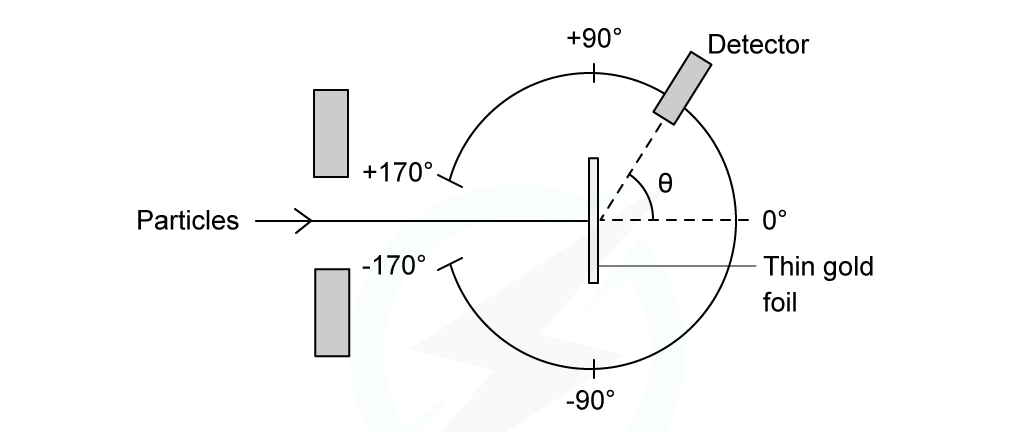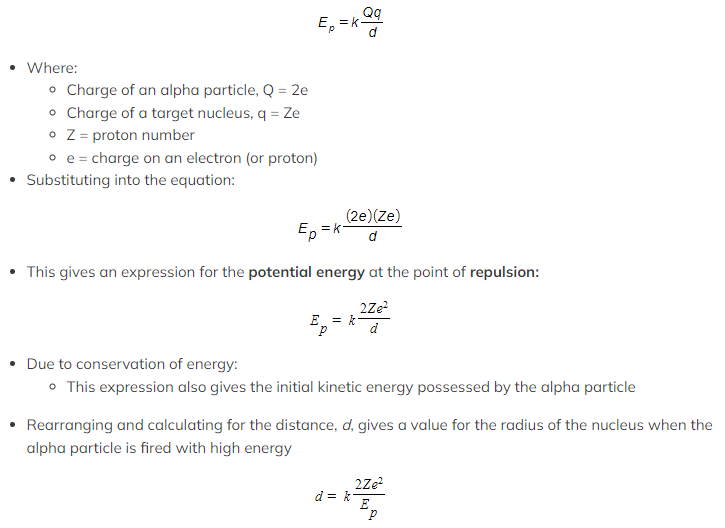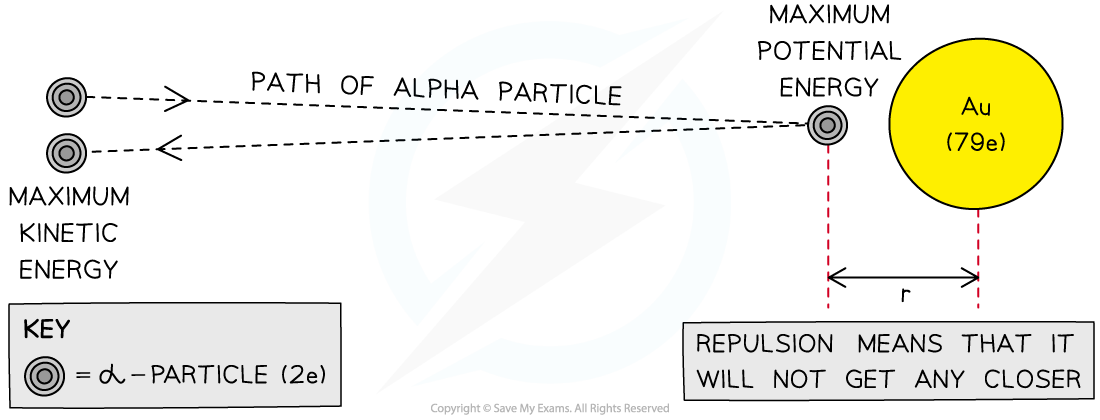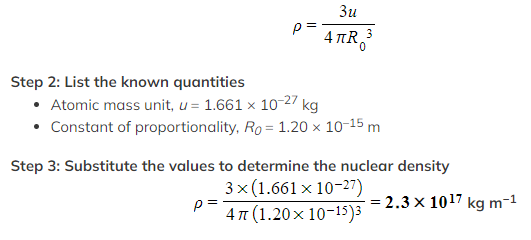# IB DP Physics: HL复习笔记12.2.1 Rutherford Scattering & Nuclear Radius

### Rutherford Scattering & Nuclear Radius

• In the Rutherford scattering experiment, alpha particles are fired at a thin gold foil
• Initially, before interacting with the foil, the particles have kinetic energy,• Some of the alpha particles are found to come straight back from the gold foil
• This indicates that there is electrostatic repulsion between the alpha particles and the gold nucleusExperimental set up of the Rutherford alpha scattering experiment

• At the point of closest approach, d, the repulsive force reduces the speed of the alpha particles to zero momentarily, before any change in direction
• At this point, the initial kinetic energy of an alpha particle, Ek, is equal to electric potential energy, Ep
• The radius of the closest approach can be found be equating the initial kinetic energy to the electric potential energyThe closest approach method of determining the size of a gold nucleus

• The radius of nuclei depends on the nucleon number, A of the atom
• This makes sense because as more nucleons are added to a nucleus, more space is occupied by the nucleus, hence giving it a larger radius
• The exact relationship between the radius and nucleon number can be determined from experimental data
• By doing this, physicists were able to deduce the following relationship:• Where:
• R = nuclear radius (m)
• A = nucleon / mass number
• R0 = constant of proportionality = 1.20 fm

#### Nuclear Density

• Assuming that the nucleus is spherical, its volume is equal to:• Where R is the nuclear radius, which is related to mass number, A, by the equation:• Where R0 is a constant of proportionality
• Combining these equations gives:• Therefore, the nuclear volume, V, is proportional to the mass of the nucleus, A
• Mass (m), volume (V), and density (ρ) are related by the equation:• The mass, m, of a nucleus is equal to:

m = Au

• Where:
• A = the mass number
• u = atomic mass unit
• Using the equations for mass and volume, nuclear density is equal to:• Since the mass number A cancels out, the remaining quantities in the equation are all constant
• Therefore, this shows the density of the nucleus is:
• Constant
• The fact that nuclear density is constant shows that nucleons are evenly separated throughout the nucleus regardless of their size
• The accuracy of nuclear density depends on the accuracy of the constant R0, as a guide nuclear density should always be of the order 1017 kg m–3
• Nuclear density is significantly larger than atomic density, this suggests:
• The majority of the atom’s mass is contained in the nucleus
• The nucleus is very small compared to the atom
• Atoms must be predominantly empty space

#### Worked Example

Determine the value of nuclear density.

You may take the constant of proportionality, R0, to be 1.20 × 10–15 m.

Step 1: Derive an expression for nuclear density

Using the equation derived above, the density of the nucleus is:#### Exam Tip

Make sure you're comfortable with the calculations involved with the alpha particle closest approach method, as this is a common exam question.
You will be expected to remember that the charge of an α is the charge of 2 protons (2 × the charge of an electron)